SBOS900B September   2018  – June 2019

PRODUCTION DATA.

1. Features
2. Applications
3. Description
1.     Device Images
4. Revision History
5. Pin Configuration and Functions
6. Specifications
7. Detailed Description
1. 7.1 Overview
2. 7.2 Functional Block Diagram
3. 7.3 Feature Description
4. 7.4 Device Functional Modes
8. Application and Implementation
1. 8.1 Application Information
2. 8.2 Typical Application
9. Power Supply Recommendations
10. 10Layout
1. 10.1 Layout Guidelines
2. 10.2 Layout Example
11. 11Device and Documentation Support
1. 11.1 Device Support
1. 11.1.1 Development Support
2. 11.2 Documentation Support
3. 11.3 Receiving Notification of Documentation Updates
4. 11.4 Community Resources
5. 11.5 Trademarks
6. 11.6 Electrostatic Discharge Caution
7. 11.7 Glossary
12. 12Mechanical, Packaging, and Orderable Information

• D|8
• DGK|8
• DGK|8

### Table 1. Table of Graphs

DESCRIPTION FIGURE
Offset Voltage Production Distribution Figure 1
Offset Voltage vs Temperature (PMOS) Figure 2
Offset Voltage vs Temperature (NMOS) Figure 3
Offset Voltage vs Power Supply Figure 4
Offset Voltage vs Common-Mode Voltage Figure 5
Offset Voltage vs Common-Mode Voltage in Transition Region Figure 6
Offset Voltage Drift Figure 7
Input Voltage Noise Spectral Density Figure 8
0.1-Hz to 10-Hz Noise Figure 9
THD+N vs Frequency Figure 10
THD+N vs Output Amplitude Figure 11
Input Bias and Offset Current vs Common-Mode Voltage Figure 12
Input Bias and Offset Current vs Temperature Figure 13
Input Bias and Offset Current vs Temperature Figure 14
Open-Loop Output Impedance vs Frequency Figure 15
Maximum Output Voltage vs Frequency Figure 16
Open-Loop Gain and Phase Vs Frequency Figure 17
Open-Loop Gain vs Temperature Figure 18
Closed-Loop Gain vs Frequency Figure 19
CMRR vs Frequency Figure 20
PSRR vs Frequency Figure 21
CMRR vs Temperature Figure 22
PSRR vs Temperature Figure 23
Positive Output Voltage vs Output Current Figure 24
Negative Output Voltage vs Output Current Figure 26
Short-Circuit Current vs Temperature Figure 25
No Phase Reversal Figure 27
Phase Margin vs Capacitive Load Figure 28
Small-Signal Overshoot vs Capacitive Load (G = –1) Figure 29
Small-Signal Overshoot vs Capacitive Load (G= +1) Figure 30
Settling Time Figure 31
Negative Overload Recovery Figure 32
Positive Overload Recovery Figure 33
Small-Signal Step Response (Noninverting) Figure 34
Small-Signal Step Response (Inverting) Figure 35
Large-Signal Step Response (Noninverting) Figure 36
Large-Signal Step Response (Inverting) Figure 37
Quiescent Current vs Supply Voltage Figure 38
Quiescent Current vs Temperature Figure 39
Channel Separation vs Frequency Figure 40
EMIRR vs Frequency Figure 41
at TA = 25°C, VS = ±18 V, VCM = VS / 2, RL = 10 kΩ connected to VS / 2, and CL = 30 pF (unless otherwise noted)TA = 25°C
Figure 1. Offset Voltage Production Distribution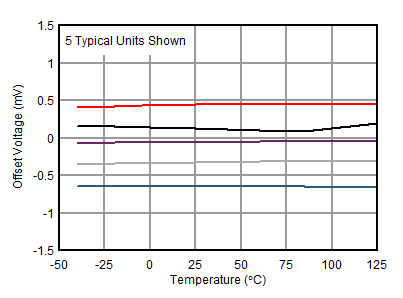NMOS region
Figure 3. Offset Voltage vs Temperature (NMOS)Figure 5. Offset Voltage vs Common-Mode Voltage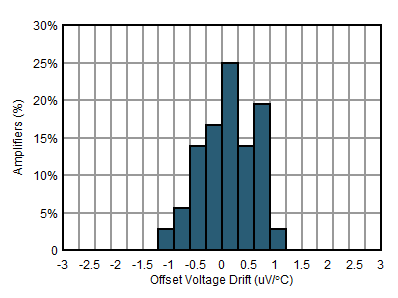TA = –40°C to +125°C
Figure 7. Offset Voltage DriftFigure 9. 0.1-Hz to 10-Hz Noise1 kHz, 80-kHz measurement bandwidth
Figure 11. THD+N vs Output Amplitude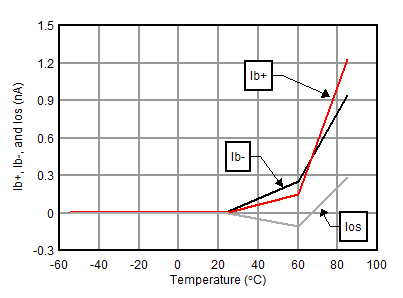TA = –55°C to +85°C
Figure 13. Input Bias and Offset Current vs Temperature (SOIC)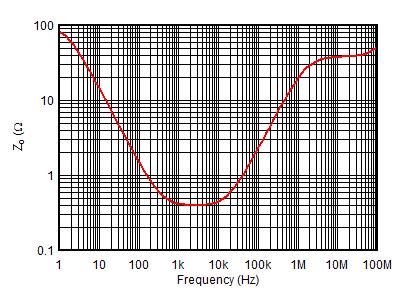Figure 15. Open-Loop Output Impedance vs FrequencyFigure 17. Open-Loop Gain and Phase vs Frequency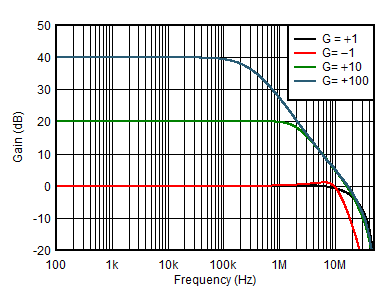Figure 19. Closed-Loop Gain vs FrequencyFigure 21. PSRR vs Frequency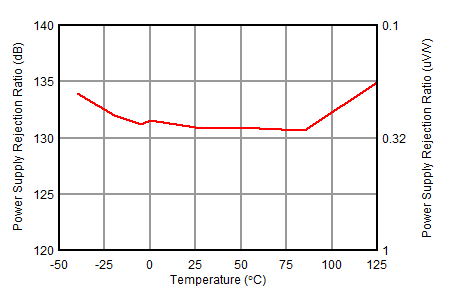Figure 23. PSRR vs Temperature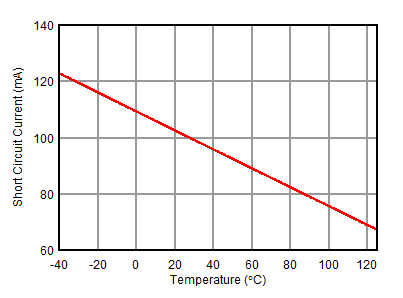Figure 25. Short-Circuit Current vs TemperatureFigure 27. No Phase Reversal10-mV output step, gain = –1
Figure 29. Small Signal Overshoot vs Capacitive LoadVin = 5-Vpp
Figure 31. Normalized Settling TimeGain = –10
Figure 33. Positive Overload Recovery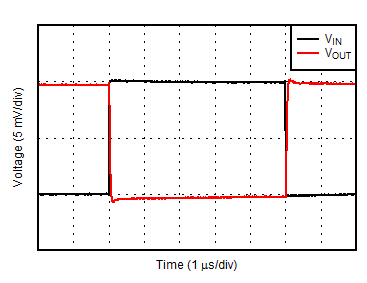Vin = 10 mVpp, gain = –1
Figure 35. Small-Signal Step Response (Inverting)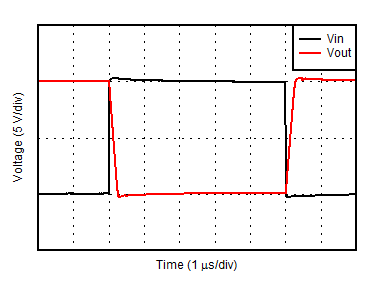Vin = 5 Vpp, gain = –1
Figure 37. Large Signal Step Response (Inverting)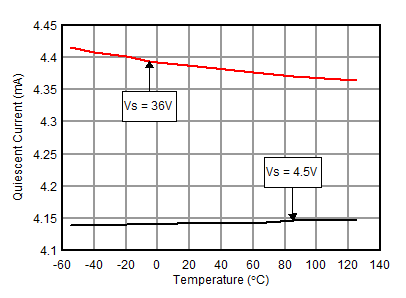Figure 39. Quiescent Current vs TemperaturePrf =-10 dBm
Figure 41. EMIRR vs FrequencyPMOS region
Figure 2. Offset Voltage vs Temperature (PMOS)Figure 4. Offset Voltage vs Power Supply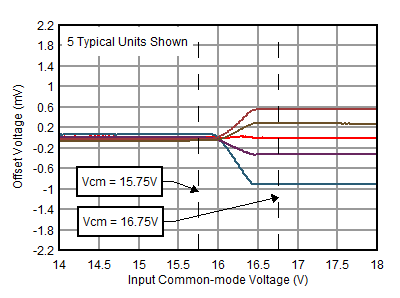Transition between PMOS and NMOS regions
Figure 6. Offset Voltage vs Common-Mode Voltage in Transition RegionFigure 8. Input Voltage Noise Spectral Density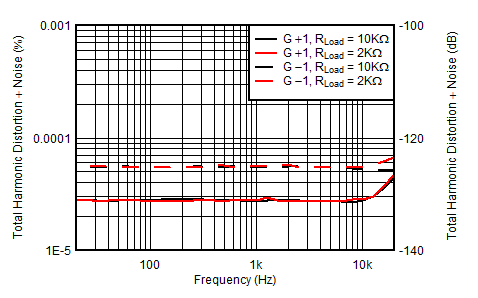3.5 VRMS, 80-kHz measurement bandwidth
Figure 10. THD+N vs FrequencyFigure 12. Input Bias and Offset Current vs Common-Mode Voltage (SOIC)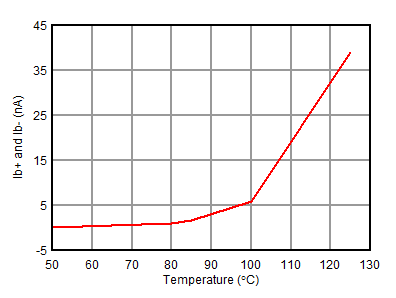TA = –55°C to +125°C
Figure 14. Input Bias and Offset Current vs Temperature (SOIC)Figure 16. Maximum Output Voltage vs Frequency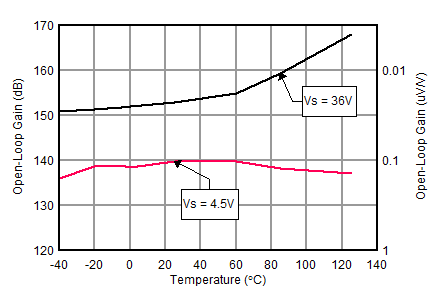Figure 18. Open-Loop Gain vs TemperatureFigure 20. CMRR vs FrequencyFigure 22. CMRR vs Temperature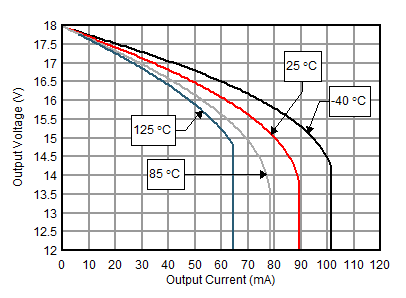Figure 24. Positive Output Voltage vs Output CurrentFigure 26. Negative Output Voltage vs Output CurrentFigure 28. Phase Margin vs Capacitive Load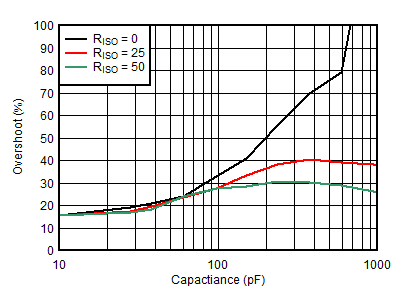10-mV output step, gain = +1
Figure 30. Small Signal Overshoot vs Capacitive LoadGain = –10
Figure 32. Negative Overload RecoveryVin = 10 mVpp, gain = 1
Figure 34. Small-Signal Step Response (Noninverting)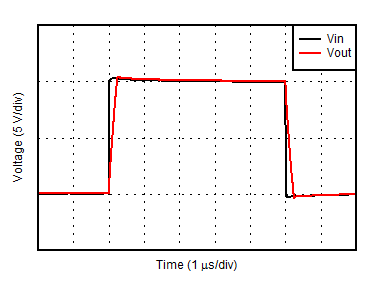Vin = 5 Vpp, gain = 1
Figure 36. Large-Signal Step Response (Noninverting)Figure 38. Quiescent Current vs Supply Voltage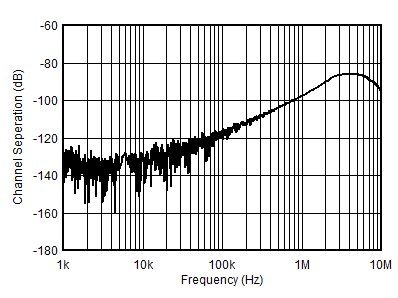Figure 40. Channel Separation vs Frequency